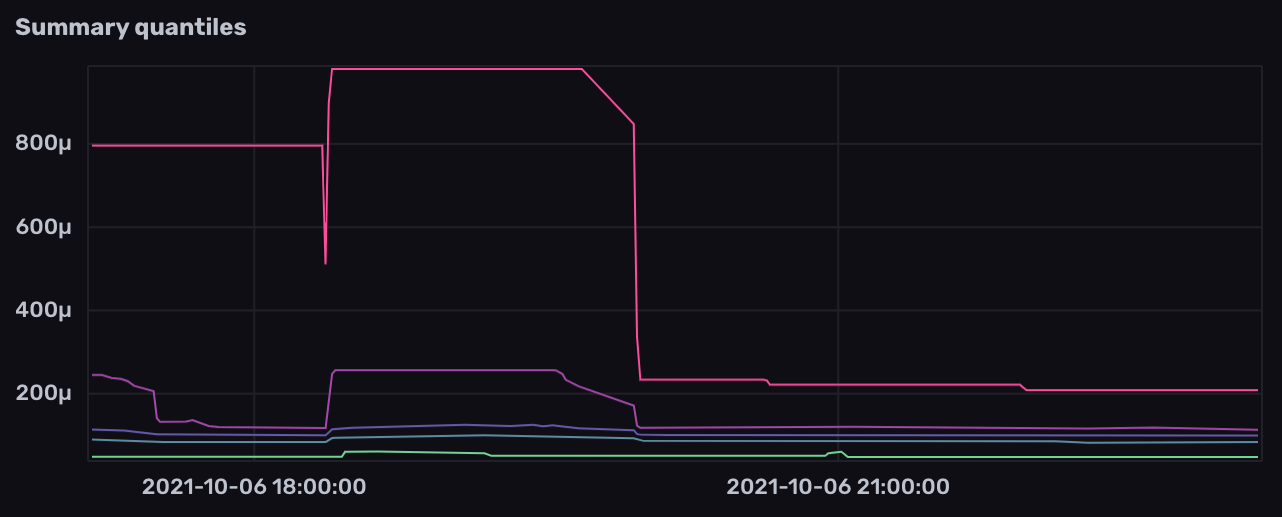# Work with Prometheus summaries

Use Flux to query and transform Prometheus summary metrics stored in InfluxDB.

A summary samples observations (usually things like request durations and response sizes). While it also provides a total count of observations and a sum of all observed values, it calculates configurable quantiles over a sliding time window.

Prometheus metric types

##### Example summary metric in Prometheus data
``````# HELP task_executor_run_duration The duration in seconds between a run starting and finishing.
example_summary_duration{label="foo",quantile="0.5"} 4.147907251
example_summary_duration{label="foo",quantile="0.9"} 4.147907251
example_summary_duration{label="foo",quantile="0.99"} 4.147907251
example_summary_duration_sum{label="foo"} 2701.367126714001
example_summary_duration_count{label="foo"} 539
``````

The examples below include example data collected from the InfluxDB OSS 2.x `/metrics` endpoint and stored in InfluxDB.

#### Prometheus metric parsing formats

Query structure depends on the Prometheus metric parsing format used to scrape the Prometheus metrics. Select the appropriate metric format version below.

## Visualize summary metric quantile values

Prometheus summary metrics provide quantile values that can be visualized without modification.

1. Filter by the `prometheus` measurement.
2. Filter by your Prometheus metric name field.
``````from(bucket: "example-bucket")
|> range(start: -1m)
|> filter(fn: (r) => r._measurement == "prometheus")
|> filter(fn: (r) => r._field == "go_gc_duration_seconds")
``````
1. Filter by your Prometheus metric name measurement.
2. Filter out the `sum` and `count` fields.
``````from(bucket: "example-bucket")
|> range(start: -1m)
|> filter(fn: (r) => r._measurement == "go_gc_duration_seconds")
|> filter(fn: (r) => r._field != "count" and r._field != "sum")
``````## Derive average values from a summary metric

Use the sum and count values provided in Prometheus summary metrics to derive an average summary value.

1. Filter by the `prometheus` measurement.
2. Filter by the `<metric_name>_count` and `<metric_name>_sum` fields.
3. Use `pivot()` to pivot fields into columns based on time. Each row then contains a `<metric_name>_count` and `<metric_name>_sum` column.
4. Divide the `<metric_name>_sum` column by the `<metric_name>_count` column to produce a new `_value`.
``````from(bucket: "example-bucket")
|> range(start: -1m)
|> filter(fn: (r) => r._measurement == "prometheus")
|> filter(fn: (r) => r._field == "go_gc_duration_seconds_count" or r._field == "go_gc_duration_seconds_sum")
|> pivot(rowKey: ["_time"], columnKey: ["_field"], valueColumn: "_value")
|> map(fn: (r) => ({r with _value: r.go_gc_duration_seconds_sum / r.go_gc_duration_seconds_count}))
``````
1. Filter by your Prometheus metric name measurement.
2. Filter by the `count` and `sum` fields.
3. Use `pivot()` to pivot fields into columns. Each row then contains a `count` and `sum` column.
4. Divide the `sum` column by the `count` column to produce a new `_value`.
``````from(bucket: "example-bucket")
|> range(start: -1m)
|> filter(fn: (r) => r._measurement == "go_gc_duration_seconds")
|> filter(fn: (r) => r._field == "count" or r._field == "sum")
|> pivot(rowKey:["_time"], columnKey: ["_field"], valueColumn: "_value")
|> map(fn: (r) => ({ r with _value: r.sum / r.count }))
``````

### Introducing InfluxDB Clustered

A highly available InfluxDB 3.0 cluster on your own infrastructure.

InfluxDB Clustered is a highly available InfluxDB 3.0 cluster built for high write and query workloads on your own infrastructure.

InfluxDB Clustered is currently in limited availability and is only available to a limited group of InfluxData customers. If interested in being part of the limited access group, please contact the InfluxData Sales team.

### The future of Flux

Flux is going into maintenance mode. You can continue using it as you currently are without any changes to your code.

Flux is going into maintenance mode and will not be supported in InfluxDB 3.0. This was a decision based on the broad demand for SQL and the continued growth and adoption of InfluxQL. We are continuing to support Flux for users in 1.x and 2.x so you can continue using it with no changes to your code. If you are interested in transitioning to InfluxDB 3.0 and want to future-proof your code, we suggest using InfluxQL.

For information about the future of Flux, see the following:

### State of the InfluxDB Cloud Serverless documentation

InfluxDB Cloud Serverless documentation is a work in progress.

The new documentation for InfluxDB Cloud Serverless is a work in progress. We are adding new information and content almost daily. Thank you for your patience!

If there is specific information you’re looking for, please submit a documentation issue.